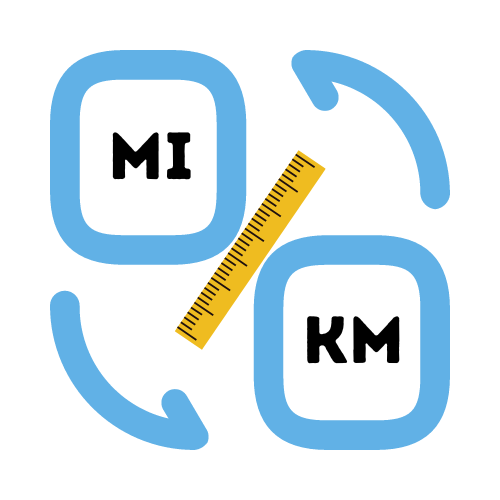# Convert Kilometers to Miles

## Simple conversion of km to miles.

• Created by Emily Rodriguez
• Reviewed by Ramesh Agarwal

Enter distance in KM

Enter a number.

## How many kilometers are in a mile?

Kilometers and miles are both units of measurement used to gauge the distance between two places. In the metric system, the size of a unit is based on how far light travels in a vacuum over all. So, how many miles to the kilometer? A kilometer is equal to 1000 meters, and a mile is equal to 1.61 kilometers. These units appear quite different in size but can still be useful when comparing distances from two different regions.

## How many miles is 5 kilometers?

While not everyone is a math expert, you may have noticed that charts are sometimes in miles but other times it will be their equivalent unit. While these equivalents are useful to determine an accurate size, they aren't always readily available when needed.

The easiest way to convert kilometers to miles is to multiply the total kilometers by 0.6214. This is easy if you have a calculator handy. For example, to calculate 5 km to miles we would do `5 * 0.6214 = 3.11 miles`.

If you need to do the calculation in your head, then you can get a rough equivalent by reducing the calculation to 2 decimal points (0.62).

## Why does america use miles?

When the English settlers first arrived in America, they brought their imperial system with them. Although the United States was different from its mother country in many ways, they continued to use the imperial system that was introduced to them.The reason why England and the United States both kept using their imperial systems was because there were standards for all things related to length and volume that remained intact throughout all of this time. This gave both countries a distinct advantage over other places around the world whose inhabitants had to learn a new way of measuring things.

In modern times there are some changes in how both countries use these systems. America is still using the imperial system to this day. However, England has moved towards a hybrid system of using the Imperial system for some measurements (ie. miles) while using the Metric system for others (ie. centimeters).

## How do you convert kilometers to miles?

Each kilometer has a distance of 0.6214 miles. A mile is quivalent to 1.60934 kilometers. Using these values, we can easily calculate the distance in either miles or kilometers. Let's use an example to see how this works.

Example conversion of km to miles:
10 kilometers to miles can be calculated as `10 * 0.6214 = 6.21 miles`.

There are several options when it comes to converting kilometers to miles. But let's first focus on what is the easiest way to convert km to miles. Probably the easiest way, is to go online and search for a km to miles conversion tool.

This is why we created a free conversion calculator for you to use. This is helpful if you wanted to convert a large number of kilometers to miles. The next option is to use a regular calculator or a pen and paper. Then to convert kilometers to miles, simply multiply the number of kilometers you have by 0.62 and this will give you an approximate distance.